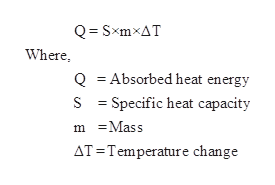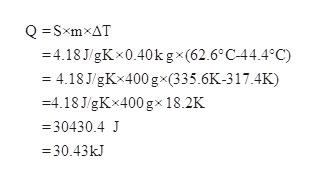# Calculate the energy required to heat 0.40kg of water from 44.4°C to 62.6°C .Assume the specific heat capacity of water under these conditions is. 4.18J·g−1K−1. Be sure your answer has the correct number of significant digits.

Question

Calculate the energy required to heat 0.40kg of water from 44.4°C to 62.6°C .Assume the specific heat capacity of water under these conditions is. 4.18J·g−1K−1. Be sure your answer has the correct number of significant digits.

check_circle

Step 1

Absorbed heat energy is given as,help_outlineImage TranscriptioncloseQ SxmxAT Where Q Absorbed heat energy S Specific heat capacity mMass AT Temperature change fullscreen
Step 2

The give values are substituted in above equation to get required energy...help_outlineImage TranscriptioncloseQSxmxAT -4.18 J/gKx0.40k gx(62.6°C44.4°C) = 4.18 J/gKx400 gx(335.6K-317.4K) =4.18J/gKx400 gx 18.2K 30430.4 J =30.43kJ fullscreen

### Want to see the full answer?

See Solution

#### Want to see this answer and more?

Solutions are written by subject experts who are available 24/7. Questions are typically answered within 1 hour.*

See Solution
*Response times may vary by subject and question.
Tagged in

### Chemical Thermodynamics